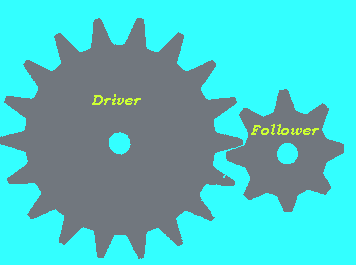Gears Gears have many uses in our lives. They are used to : - multiply or reduce speed and force; - change the direction of motion; - transmit a force over a distance. Intermeshing gears are used to transmit motion and force. A series of intermeshing gears is called a gear train. Intermeshing gears turn in opposing directions. An understanding of some of the terminology is needed. In a gear train we have a gear known as the driver and one known as the follower. Driver - is the gear that has the force or motion input. Follower - is the gear that results in the force or motion output.The above diagram shows a very simple gear train. Notice how the gears spin in opposite directions. If we were to turn the large gear (driver) we can multiply the number of turns generated by the smaller gear (follower). We often talk about a gear ratio. The gear ratio of a gear train is the number of teeth on the follower divided by the number of teeth on the driver. In the gear train above the driver has 18 teeth while the follower has 8 teeth. Therefore the gear ratio is 8/18 or 4/9. For every 4 turns of the driver the follower turns 9 times. This gear train can be used to multiply speed on a bicycle if the follower was connected to a wheel and the driver connected to the paddles. Now if we were to change the driver and the follower we can increase the gear ratio. The gear ratio of the gear train on the right is 18/8 or 9/4. For every 9 turns of the driver the follower will make 4 complete rotations. Now this gear train is not for multiplying speed but for multiplying force.A simple rule applies. Low gear ratio = more speed = less force High gear ratio= less speed = more force. Continue

Home